Online Tution   »   Important Question   »   CBSE Class 12 Mathematics Term 2...

# CBSE Class 12 Maths Term 2 Additional Practice Questions 2022

## CBSE Class 12 Maths Term 2 Additional Practice Questions

The students must solve all the CBSE Class 12 Mathematics Term 2 Practice Questions 2022 given on this page to get good marks in Mathematics. Mathematics is all about problem-solving, the more you practice Mathematics questions the more you will become familiar with all the types of questions and it will help you get good marks in Mathematics. We have provided the CBSE Class 12 Mathematics Term 2 Practice Questions 2022 on this page. The students appearing in CBSE Term 2 Exam 2022 must bookmark this page to get all the updates from CBSE.

## CBSE Class 12 Maths Additional Term 2 Practice Questions

CBSE Term 2 Mathematics holds 40 marks and students will be given 2 hours to complete the question paper. The students can increase their problem-solving speed by solving the CBSE Class 12 Mathematics Term 2 Practice Questions 2022 given on this page. The CBSE will conduct CBSE Class 12 Term 2 Exam 2022 between 26th April to 15th June 2022. The students can consolidate their preparation for Mathematics by solving the CBSE Class 12 Mathematics Term 2 Practice Questions 2022.

## CBSE Class 12th Maths Term 2 Practice Additional Questions: Exam Pattern

Students appearing in the CBSE Class 12 Term 2 Exam 2022 must be familiar with the CBSE Term 2 exam pattern. CBSE Term 2 Exam 2022 is subjective, therefore students must prepare differently for the CBSE Term 2 exam. The exam pattern for Mathematics is based on the sample paper released by the board on its official website. Check the Section-wise exam pattern listed below:

Section A: This section has very short type questions of 2 marks each.

Section B: This section has short-type questions of 3 marks each.

Section C: This section has long-type questions of 4 marks each.

## CBSE Class 12th Maths Term 2 Additional Practice Questions

1. Study the equation given below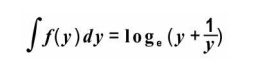OR

Integrate: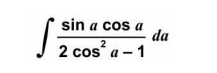2. Shown below is a differential equation.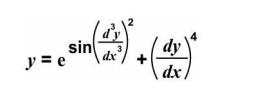Find the order and the degree of the given differential equation. Give reasons to support your answer.

3. Shown below are two vectors in their component forms.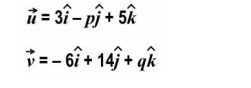For what values of p and q are the vectors collinear? Show your steps.

4. A(-1, 3, 2), B(-2, 3, -1), C(-5, -4, p) and D(-2, -4, 3) are four points in space. Lines AB and CD are parallel. Find the value of p. Show your work and give valid reasons.

5. Bhavani is going to play a game of chess against one of four opponents in an intercollege sports competition. Each opponent is equally likely to be paired against her. The table below shows the chances of Bhavani losing, when paired against each opponent.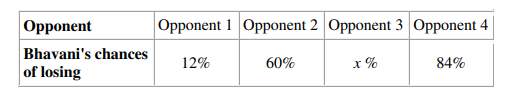If the probability that Bhavani loses the game that day is 1/2, find the probability for Bhavani to be losing the game when paired against Opponent 3. Show your steps.

6. In the game of archery, the probability of Likith and Harish hitting the target are 2/3 and 3/4 respectively. If both of them shoot an arrow, find the probability that the target is NOT hit by either of them. Show your steps.

SECTION B

7. The first derivative of a function f(x) is given below.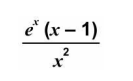If f(1) = e + 3, find f(x). Show your steps.

8. Find the general solution of the differential equation given below. Show your steps.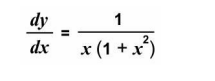OR
Shown below is a differential equation where the value of y is 0 when x = 3.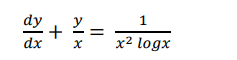Find the value of y when x = 5. Show your steps.

9. Three vertices – A, B and D of a parallelogram ABCD are given by, A(0, -3, 3), B(-5, m – 3, 0) and D(1, -3, 4). The area of the parallelogram ABCD is 6 sq units. Using the vector method, find the value(s) of m. Show your steps.

OR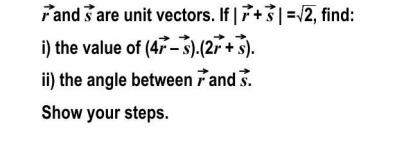10. The vector form of equations of two lines, l1 and l2 are: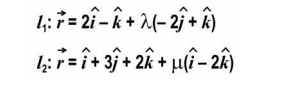Show that l1 and l2 are skew lines.

SECTION C

11. Evaluate: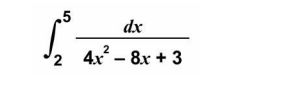12. Shown below is a parabola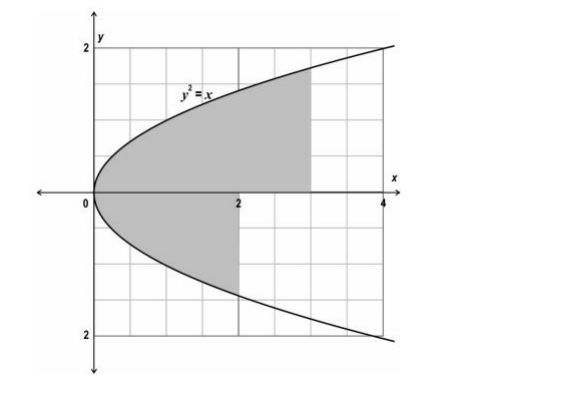(Note: Take √2 as 1.4 and √3 as 1.7.)
OR
Shown below is the graph of f(x) = 2×2 in the first quadrant.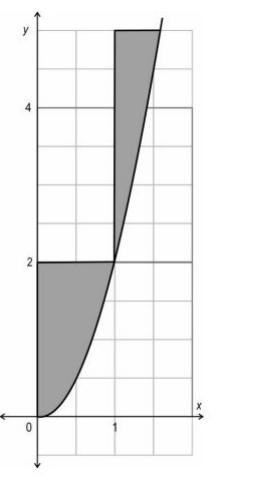(Note: You need not evaluate the square roots.)

13. Shown below are equations of two planes.
Plane 1: −6𝑥 − 3𝑦 − 2𝑧 = 12
Plane 2: 𝑟⃗(2𝑖̂ − 11𝑗̂ − 10𝑘̂) − 6 = 0
Is the point (-2, -3, -1) closer to plane 1 or plane 2? Show your work.

14. Read the information given below and answer the questions that follow. For an audition for a reality singing competition, interested candidates were asked to apply under one of the two musical genres – folk or classical and under one of the two age categories – below 18 or 18 and above. The following information is known about the 2000 applications received:
♦ 960 of the total applications were for the folk genre.
♦ 192 of the folk applications were for the below 18 category.
♦ 104 of the classical applications were for the 18 and above category.
a) What is the probability that an application selected at random is for the 18 and above category provided it is under the classical genre? Show your work.
b) An application selected at random is found to be under the below 18 category. Find the probability that it is under the folk genre. Show your work.

## FAQs on CBSE Class 12 Mathematics Term 2 Additional Practice Questions 2022

Q. What is the CBSE Class 12 Term 2 Exam Date 2022?

CBSE is all set to conduct CBSE Term 2 Exam 2022. The CBSE Class 12 Term 2 Exam 2022 will begin on 26th April 2022 and will be continued till 15th June 2022.

Q. Where I can get CBSE Class 12 Mathematics Term 2 Practice Questions 2022?

We have given CBSE Class 12 Mathematics Term 2 Practice Questions 2022 on this page. For other subjects, the students can visit the official website of Adda247 School.

Q. What is the CBSE Term 2 Mathematics Exam Pattern 2022?

Mathematics holds 40 marks and students will get 2 hours to solve the question paper. The Term 2 Mathematics question paper is divided into three sections. Section A carries 2 marks questions, Section B carries 3 marks questions and Section C carries 4 marks questions.

Sharing is caring!

Thank You, Your details have been submitted we will get back to you.